Next: Time-Dependent Perturbation Theory Up: Time-Independent Perturbation Theory Previous: Zeeman Effect

# Hyperfine Structure

The proton in a hydrogen atom is a spin one-half charged particle, and therefore possesses a magnetic moment. By analogy with Eq. (759), we can write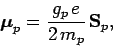(1004)

where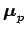is the proton magnetic moment,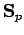is the proton spin, and the proton gyromagnetic ratiois found experimentally to take that value. Note that the magnetic moment of a proton is much smaller (by a factor of order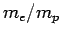) than that of an electron. According to classical electromagnetism, the proton's magnetic moment generates a magnetic field of the form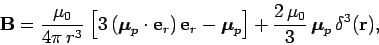(1005)

where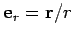. We can understand the origin of the delta-function term in the above expression by thinking of the proton as a tiny current loop centred on the origin. All magnetic field-lines generated by the loop must pass through the loop. Hence, if the size of the loop goes to zero then the field will be infinite at the origin, and this contribution is what is reflected by the delta-function term. Now, the Hamiltonian of the electron in the magnetic field generated by the proton is simply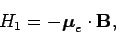(1006)

where(1007)

Here,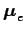is the electron magnetic moment [see Eqs. (759) and (760)], and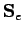the electron spin. Thus, the perturbing Hamiltonian is written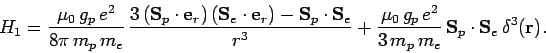(1008)

Note that, since we have neglected coupling between the proton spin and the magnetic field generated by the electron's orbital motion, the above expression is only valid for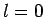states.

According to standard first-order perturbation theory, the energy-shift induced by spin-spin coupling between the proton and the electron is the expectation value of the perturbing Hamiltonian. Hence,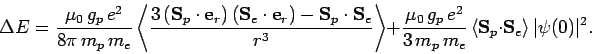(1009)

For the ground-state of hydrogen, which is spherically symmetric, the first term in the above expression vanishes by symmetry. Moreover, it is easily demonstrated that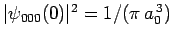. Thus, we obtain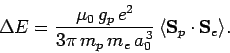(1010)

Let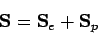(1011)

be the total spin. We can show that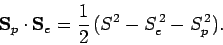(1012)

Thus, the simultaneous eigenstates of the perturbing Hamiltonian and the main Hamiltonian are the simultaneous eigenstates of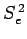,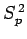, and. However, both the proton and the electron are spin one-half particles. According to Sect. 11.4, when two spin one-half particles are combined (in the absence of orbital angular momentum) the net state has either spin 1 or spin 0. In fact, there are three spin 1 states, known as triplet states, and a single spin 0 state, known as the singlet state. For all states, the eigenvalues ofandare. The eigenvalue ofis 0 for the singlet state, andfor the triplet states. Hence,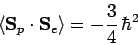(1013)

for the singlet state, and(1014)

for the triplet states.

It follows, from the above analysis, that spin-spin coupling breaks the degeneracy of the twostates in hydrogen, lifting the energy of the triplet configuration, and lowering that of the singlet. This splitting is known as hyperfine structure. The net energy difference between the singlet and the triplet states is(1015)

whereis the (magnitude of the) ground-state energy. Note that the hyperfine energy-shift is much smaller, by a factor, than a typical fine structure energy-shift. If we convert the above energy into a wavelength then we obtain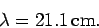(1016)

This is the wavelength of the radiation emitted by a hydrogen atom which is collisionally excited from the singlet to the triplet state, and then decays back to the lower energy singlet state. The 21cm line is famous in radio astronomy because it was used to map out the spiral structure of our galaxy in the 1950's.Next: Time-Dependent Perturbation Theory Up: Time-Independent Perturbation Theory Previous: Zeeman Effect
Richard Fitzpatrick 2010-07-20